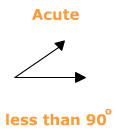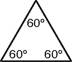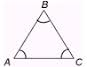Classifying Triangles by Angles

There are two ways to classify triangles. To classify a triangle by angles means to categorize the triangle according to the types of angles that make up the triangle. To classify triangles by angles you must determine if each angle in the triangle is acute, right or obtuse. Once you have determined the types of angles you can classify the triangle as an Acute Triangle, Right Triangle, or Obtuse Triangle.

Let's review three different types of angles.Now let's look at how that works with triangles.Let's look at triangles with angle measures.If all three angles are the same measure (60°) then the triangle is called EQUIANGULAR.

It will look like one of the two examples below.Let's review.

• If all the angles of the triangle are all less than 90° then the triangle is classified as an ACUTE TRIANGLE.

• If all the angles in the triangle are 60° or if they are all marked as congruent then the triangle is an ACUTE TRIANGLE but it is also a special triangle called an EQUIANGULAR triangle.

• If one of the angles is 90° then the triangle is a RIGHT TRIANGLE.

• If one of the angles is greater than 90° then the triangle is classified as an OBTUSE TRIANGLE.

 Related Links: Math Geometry Topics Classifying Triangles by Sides

To link to this Classifying Triangles by Angles page, copy the following code to your site: